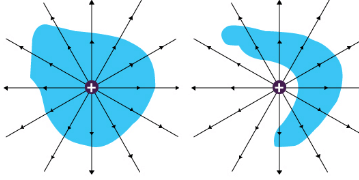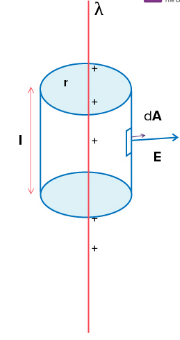The Gauss Law

# The Gauss Law - Class 12

What is guass law and how to apply guassian surface?
Ref: https://edurev.in/question/663740/What-is-guass-law-and-how-to-apply-guassian-surface-

The Gauss law is a very convenient tool to find the electric field of a system of charges. It is especially useful when situations of symmetry can be easily exploited. The electric flux (dΦ) through a differential area is the dot product of the electric field and the area vector (this vector is normal to the area pointing towards the convex end; its magnitude is the area magnitude).

dΦ = →E . d→A = EdA cos θ

Where θ is the angle between the electric field and the area vector

The net flux through a surface is simply the integral of the differential flux dΦ,

Φ = ∫ dΦ = ∫ →E.d→A

For a closed surface such as that of a sphere, torus or a cube, this integral is represented as a loop integral

Φ = →E.d→A

The Gauss Theorem

The net flux through a closed surface is directly proportional to the net charge in the volume enclosed by the closed surface.

Φ = →E.d→A = qnet/ε0

In simple words, the Gauss law relates the ‘flow’ of electric field lines (flux) to the charges within the enclosed surface. If there are no charges enclosed by a surface, then the net electric flux remains zero. This means that the number of electric field lines entering the surface is equal to the field lines leaving the surface.

This statement also gives an important corollary: the electric flux from any closed surface is only due to the sources (positive charges) and sinks (negative charges) of electric fields enclosed by the surface. Any charges outside the surface do not contribute to the electric flux. Also, only electric charges can act as sources or sinks of electric fields. Changing magnetic fields. For example, cannot act as sources or sinks of electric fields.The net flux for the surface on the left is non-zero as it encloses a net charge. The net flux for the surface on the right is zero since it does not enclose any charge.

Note that the Gauss Law is only a restatement of the Coulomb’s Law. If you apply Gauss Law to a point charge enclosed by a sphere, you will get back the Coulomb’s Law easily.

Application to an infinitely long line of charge:

Consider an infinitely long line of charge with the charge per unit length being λ. We can take advantage of the cylindrical symmetry of this situation. By symmetry, The electric fields all point radially away from the line of charge, There is no component parallel to the line of charge.

We can use a cylinder (with any arbitrary radius r and length l) centered on the line of charge as our Gaussian surface.As you can see in the above diagram, the electric field is perpendicular to the curved surface (hence parallel to the area vector) of the cylinder. Thus, the angle between the electric field and area vector is zero and cos θ = 1

The top and bottom surfaces of the cylinder lie parallel to the electric field. Thus the angle between area vector and electric field is 90 degrees and cos θ = 0.

Thus the electric flux is only due to the curved surface:

Φ = →E.d→A

Φ = Φcurved + Φtop + Φbottom

Φ = →E.d→A = ∫ EdA cos 0 + ∫ EdA cos 90° + ∫ EdA cos 90°

Φ = ∫ EdA.1

Due to radial symmetry, the curved surface is equidistant from the line of charge and the electric ield in the surface has a constant magnitude throughout.

Φ = ∫ EdA = E ∫ dA = E.2πrl

The net charge enclosed by the surface is:

qnet = λ.l

Using Gauss theorem,

Φ = E.2πrl = qnet/ε0 = λ.l/ε0

E.2πrl = λ.l/ε0

E = 1/2πε0 λ/r

The electric field, in this case, falls off only by the inverse of r. Log on to www.byjus.com for more interesting articles.

The document The Gauss Law - Class 12 is a part of Class 12 category.
All you need of Class 12 at this link: Class 12

## FAQs on The Gauss Law - Class 12

 1. What is Gauss's law in physics?Ans. Gauss's law is a fundamental principle in physics that relates the electric flux through a closed surface to the net electric charge enclosed within the surface. It states that the electric flux through any closed surface is proportional to the total electric charge enclosed by that surface divided by the permittivity of the medium.
 2. How is Gauss's law mathematically expressed?Ans. Mathematically, Gauss's law is expressed as ∮E⋅dA = Q/ε₀, where ∮E⋅dA represents the electric flux through a closed surface, Q is the total electric charge enclosed within the surface, and ε₀ is the permittivity of the medium.
 3. What is the significance of Gauss's law in electromagnetism?Ans. Gauss's law is significant in electromagnetism as it provides a powerful tool to calculate the electric field generated by various charge distributions. It helps in understanding the behavior of electric charges and their effect on the surrounding electric field. It also allows us to calculate the net electric charge enclosed within a closed surface based on the electric flux through that surface.
 4. Can Gauss's law be applied to any closed surface?Ans. Gauss's law can be applied to any closed surface, irrespective of its shape or size. The surface can be chosen in a way that simplifies the calculations of the electric flux or makes use of symmetry to analyze the electric field. By appropriately selecting the closed surface, Gauss's law can be used to determine the electric field due to various charge distributions.
 5. How is Gauss's law related to Coulomb's law?Ans. Gauss's law and Coulomb's law are closely related. Coulomb's law gives the electric field due to a point charge, while Gauss's law allows us to calculate the electric field due to various charge distributions. By applying Gauss's law to a closed surface surrounding a point charge, we can derive Coulomb's law. Gauss's law provides a more general framework to analyze electric fields, while Coulomb's law is a specific case of Gauss's law for a point charge.
Signup to see your scores go up within 7 days! Learn & Practice with 1000+ FREE Notes, Videos & Tests.
10M+ students study on EduRev
Track your progress, build streaks, highlight & save important lessons and more!(Scan QR code)
Related Searches

,

,

,

,

,

,

,

,

,

,

,

,

,

,

,

,

,

,

,

,

,

;